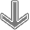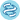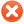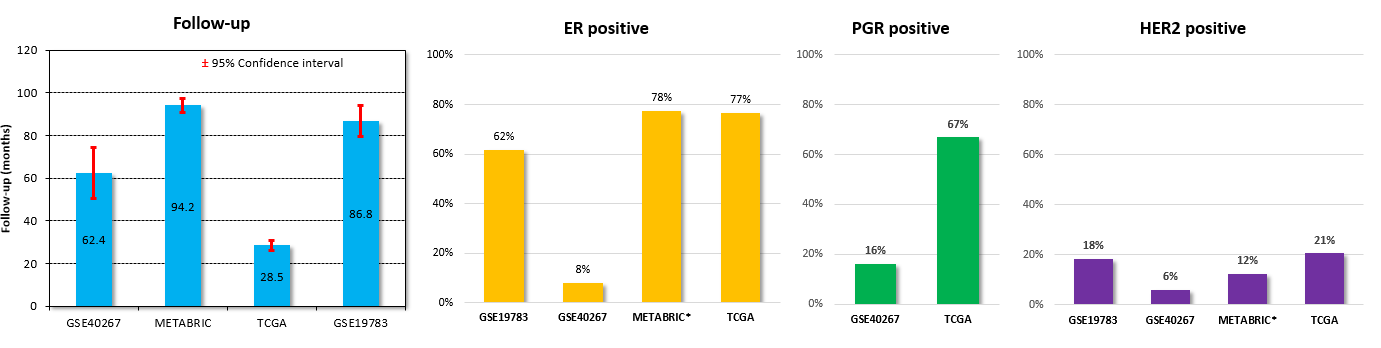Plot options1. Select gene symbol/Uniprot ID:2. Select dataset:

 Liu_2014 (n=126) TCGA-RPPA (n=873) DeMarchi_2015 (n=112) Tang_2018 (n=118)

## Survival options

 Survival OS (n=1064)RFS (n=986)DMFS (n=126)Split patients by --none--lower quartilelower tertilemedianupper tertileupper quartile Auto select best cutoffTrichotomization : --none--T1 vs T3Q1 vs Q4Follow up threshold: all2 months4 months6 months12 months18 months24 months30 months36 months48 months60 months120 months180 months240 monthsCensore at threshold:Compute median survival:Export plot data as text: Exclude samples with a protein expression of 0:## Restrict analysis to subtypes...

 ER status: (n=1126) all each separately positive (n=771) negative (n=355) PGR status: (n=946) all each separately positive (n=532) negative (n=414) HER2 status: (n=704) all each separately positive (n=133) negative (n=571)

 Stage: (n=923) all each separately 1 (n=134) 2 (n=551) 3 (n=220) 4 (n=18) Grade: (n=160) all each separately 1 (n=10) 2 (n=35) 3 (n=115) Lymph node status: (n=1153) all each separately positive (n=522) negative (n=631)

 T: (n=984) all each separately 1 (n=233) 2 (n=593) 3 (n=122) 4 (n=36) N: (n=859) all each separately no reg.ln.met. (n=407) reg.ln.met. (n=287) tumor spread between N1 and N3 (n=103) tumour spread to distant or numerous reg. ln. (n=62) M: (n=757) all each separately no metastasis (n=737) metastasis (n=20)

## Restrict analysis to selected cohorts...

 Race: (n=930) all each separately white (n=682) asian (n=58) black/african american (n=190) Menopausal status: (n=125) all each separately pre (n=59) post (n=66)

 Radiation therapy: (n=122) all 0 (n=69) 1 (n=53) Hormone therapy: (n=1111) all each separately any (n=1111) Tamoxifen (n=315) Anastrozole (n=60) Chemotherapy: (n=999) all each separately any (n=999) Doxorubicin (n=307) 5-FU (n=84) Docetaxel (n=150) Cyclophosphamide (n=410) Paclitaxel (n=188) Carboplatin (n=23)

# Compare datasetsMETABRIC: long follow-up (median: 94 months), average characteristics (78% ER positive, 12% HER2 positive), treatment data available for this dataset only!
TCGA: very short follow-up (median only 25 months), no grade.
GSE40267: almost exclusively ER negative patients (92%).
GSE19783: nodal status not available, 38% ER negative, high proportion of HER2 positive patients (18%).Please note: the generated p value does not include correction for multiple hypothesis testing by default.Please kindly cite our paper to support further development: Osz A, Lanczky A, Gyorffy B: Survival analysis in breast cancer using proteomic data from four independent datasets, Sci Rep 2021;11(1):16787.